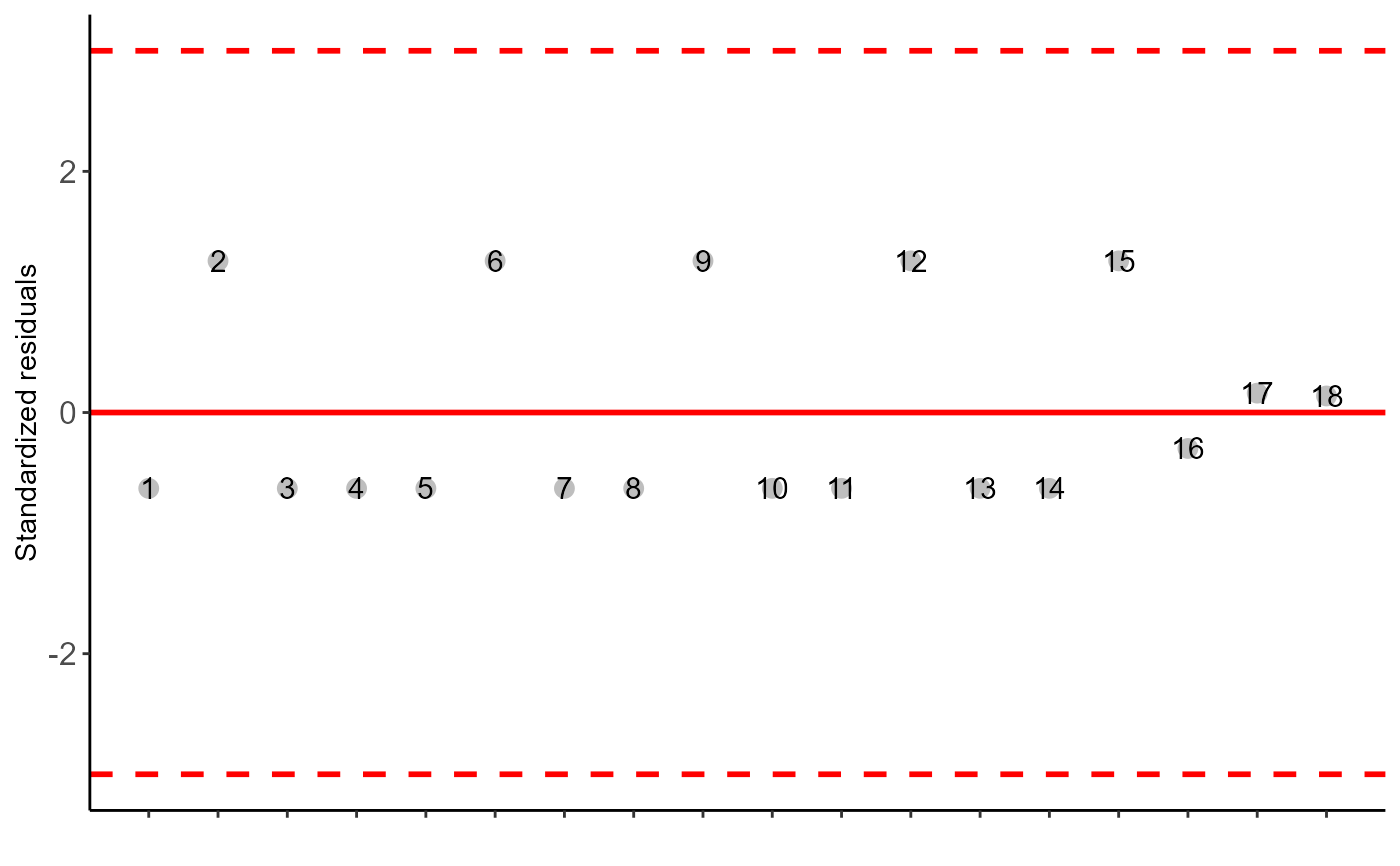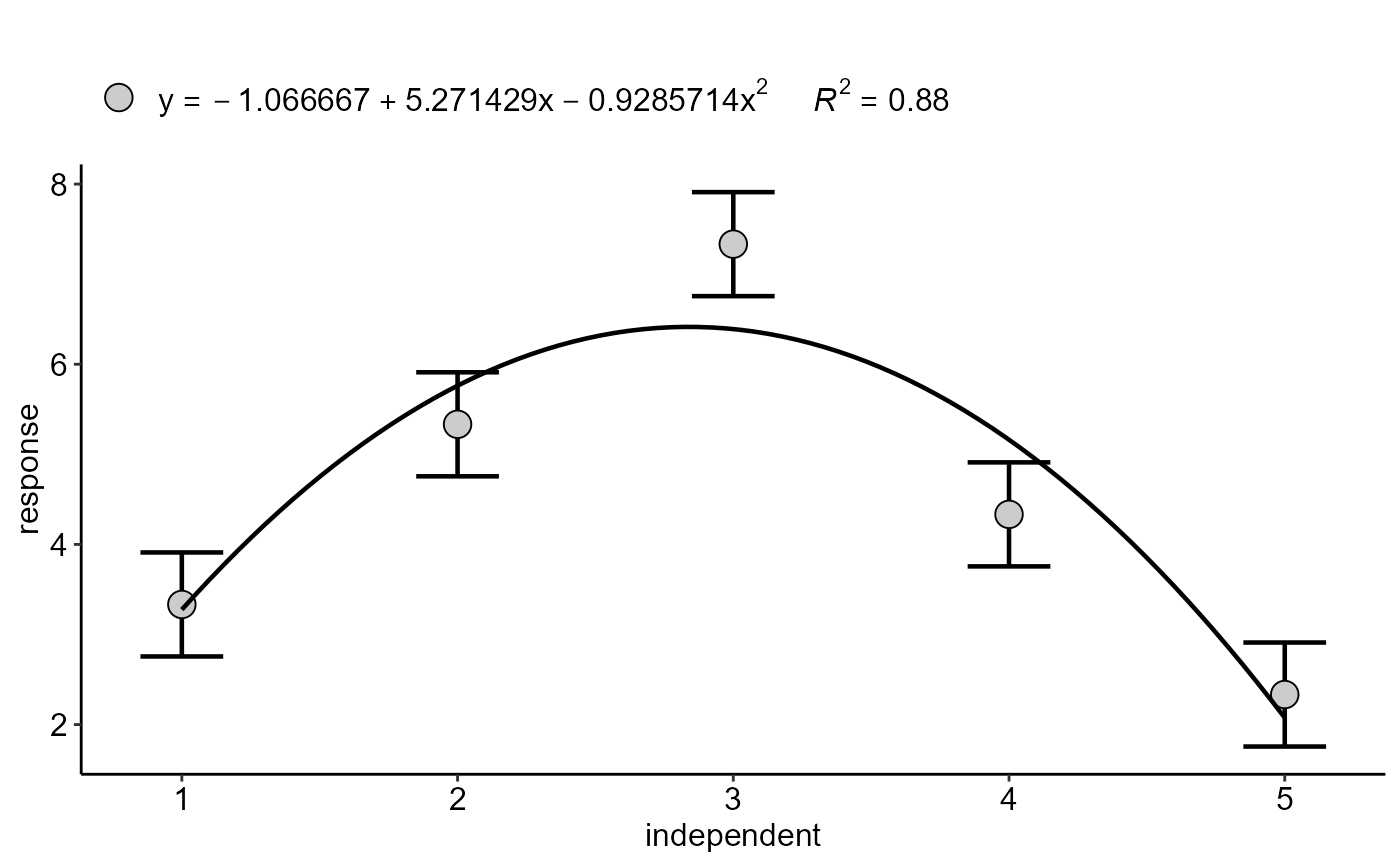Statistical analysis of experiments conducted in a completely randomized with an additional treatment and balanced design with a factor considering the fixed model.

dic.ad(
trat,
response,
grau = 1,
norm = "sw",
homog = "bt",
alpha.f = 0.05,
theme = theme_classic(),
ylab = "response",
xlab = "independent",
family = "sans",
posi = "top",
pointsize = 4.5,
linesize = 0.8,
width.bar = NA,
point = "mean_sd"
)

## Arguments

trat

Numerical or complex vector with treatments

response

Numerical vector containing the response of the experiment.

Numerical vector with additional treatment responses

grau

Degree of polynomial in case of quantitative factor (default is 1)

norm

Error normality test (default is Shapiro-Wilk)

homog

Homogeneity test of variances (default is Bartlett)

alpha.f

Level of significance of the F test (default is 0.05)

theme

ggplot2 theme (default is theme_classic())

ylab

Variable response name (Accepts the expression() function)

xlab

Treatments name (Accepts the expression() function)

family

Font family

posi

Legend position

pointsize

Point size

linesize

line size (Trendline and Error Bar)

width.bar

width of the error bars of a regression graph.

point

Defines whether to plot mean ("mean"), mean with standard deviation ("mean_sd" - default) or mean with standard error (default - "mean_se"). For quali=FALSE or quali=TRUE.

## Value

The table of analysis of variance, the test of normality of errors (Shapiro-Wilk ("sw"), Lilliefors ("li"), Anderson-Darling ("ad"), Cramer-von Mises ("cvm"), Pearson ("pearson") and Shapiro-Francia ("sf")), the test of homogeneity of variances (Bartlett ("bt") or Levene ("levene")), the test of independence of Durbin-Watson errors, adjustment of regression models up to grade 3 polynomial. The function also returns a standardized residual plot.

## Note

In some experiments, the researcher may study a quantitative factor, such as fertilizer doses, and present a control, such as a reference fertilizer, treated as a qualitative control. In these cases, there is a difference between considering only the residue in the unfolding of the polynomial, removing or not the qualitative treatment, or since a treatment is excluded from the analysis. In this approach, the residue used is also considering the qualitative treatment, a method similar to the factorial scheme with additional control.

## Author

Gabriel Danilo Shimizu, shimizu@uel.br

Leandro Simoes Azeredo Goncalves

Rodrigo Yudi Palhaci Marubayashi

## Examples

datadicad=data.frame(doses = c(rep(c(1:5),e=3)),
resp = c(3,4,3,5,5,6,7,7,8,4,4,5,2,2,3))
#>
#> -----------------------------------------------------------------
#> Normality of errors
#> -----------------------------------------------------------------
#>                          Method Statistic      p.value
#>  Shapiro-Wilk normality test(W)   0.68831 5.858208e-05
#>
#> As the calculated p-value is less than the 5% significance level, H0 is rejected. Therefore, errors do not follow a normal distribution
#>
#> -----------------------------------------------------------------
#> Homogeneity of Variances
#> -----------------------------------------------------------------
#>                               Method Statistic p.value
#>  Bartlett test(Bartlett's K-squared)  3.099624 0.68463
#>
#> As the calculated p-value is greater than the 5% significance level,hypothesis H0 is not rejected. Therefore, the variances can be considered homogeneous
#>
#> -----------------------------------------------------------------
#> Independence from errors
#> -----------------------------------------------------------------
#>                  Method Statistic p.value
#>  Durbin-Watson test(DW)  2.889275 0.80449
#>
#> As the calculated p-value is greater than the 5% significance level, hypothesis H0 is not rejected. Therefore, errors can be considered independent#>
#> -----------------------------------------------------------------
#> -----------------------------------------------------------------
#>
#> CV (%) =  11.69
#> Mean =  4.5333
#> Median =  4
#> Possible outliers =  No discrepant point
#>
#> -----------------------------------------------------------------
#> Analysis of Variance
#> -----------------------------------------------------------------
#> Analysis of Variance Table
#>
#> Response: response
#>                   Df Sum Sq Mean Sq F value    Pr(>F)
#> Factor             4 44.400 11.1000  39.516 8.118e-07 ***
#> Factor vs control  1  4.969  4.9686  17.688  0.001219 **
#> Residuals         12  3.371  0.2809
#> ---
#> Signif. codes:  0 '***' 0.001 '**' 0.01 '*' 0.05 '.' 0.1 ' ' 1
#>
#> ----------------------------------------------------
#> Regression Models
#> ----------------------------------------------------
#>               Estimate Std. Error   t value     Pr(>|t|)
#> (Intercept) -1.0666667  1.0615452 -1.004824 3.348137e-01
#> trat         5.2714286  0.8089681  6.516238 2.867473e-05
#> I(trat^2)   -0.9285714  0.1322804 -7.019719 1.395317e-05
#>
#> ----------------------------------------------------
#> Deviations from regression
#> ----------------------------------------------------
#>           Df       SSq        MSQ          F      p-value
#> Linear     1  2.700000  2.7000000   9.611869 9.184851e-03
#> Quadratic  1 36.214286 36.2142857 128.921099 8.933502e-08
#> Deviation  2  5.485714  2.7428571   9.764438 3.039740e-03
#> Residual  12  3.370832  0.2809027